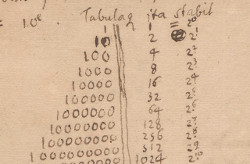# Bitmasks, bitsets and flags

yourbasic.org/golang## Bitmask

A bitmask is a small set of booleans, often called flags, represented by the bits in a single number.

``````type Bits uint8

const (
F0 Bits = 1 << iota
F1
F2
)

func Set(b, flag Bits) Bits    { return b | flag }
func Clear(b, flag Bits) Bits  { return b &^ flag }
func Toggle(b, flag Bits) Bits { return b ^ flag }
func Has(b, flag Bits) bool    { return b&flag != 0 }

func main() {
var b Bits
b = Set(b, F0)
b = Toggle(b, F2)
for i, flag := range []Bits{F0, F1, F2} {
fmt.Println(i, Has(b, flag))
}
}``````
``````0 true
1 false
2 true``````

## Larger bitsets

To represent larger sets of bits, you may want to use a custom data structure. The package `github.com/yourbasic/bit` provides a bit array implementation and some utility bit functions.

Because a bit array uses bit-level parallelism, limits memory access, and efficiently uses the data cache, it often outperforms other data structures. Here is an example that shows how to create the set of all primes less than n in O(n log log n) time using the `bit.Set` data structure from package `bit`. Try the code with n equal to a few hundred millions and be pleasantly surprised.

``````// Sieve of Eratosthenes
const n = 50
sieve := bit.New().AddRange(2, n)
sqrtN := int(math.Sqrt(n))
for p := 2; p <= sqrtN; p = sieve.Next(p) {
for k := p * p; k < n; k += p {
sieve.Delete(k)
}
}
fmt.Println(sieve)
``````
``{2 3 5 7 11 13 17 19 23 29 31 37 41 43 47}``

### Further readingBitwise operators cheat sheet

### More code examplesGo blueprints: code for com­mon tasks is a collection of handy code examples.